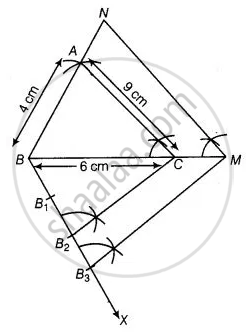# Draw a triangle ABC in which AB = 4 cm, BC = 6 cm and AC = 9 cm. Construct a triangle similar to ∆ABC with scale factor 32. Justify the construction. Are the two triangles congruent? Note that - Mathematics

Diagram
Sum

Draw a triangle ABC in which AB = 4 cm, BC = 6 cm and AC = 9 cm. Construct a triangle similar to ∆ABC with scale factor 3/2. Justify the construction. Are the two triangles congruent? Note that all the three angles and two sides of the two triangles are equal.

#### Solution

Steps of construction

1. Draw a line segment BC = 6 cm.

2. Taking B and C as centres, draw two arcs of radii 4 cm and 9 cm intersecting each other at A.

3. Join BA and CA, ΔABC is the required triangle.

4. From B, draw any ray BX downwards making an acute angle.

5. Mark three points B1, B2, B3 on BX, such that BB1 = B1B2 = B2B3.6. Join B2C and from B3 draw B3M || B2C intersecting the extended line segment BC at

7. From point M, draw MN || CA intersecting the extended line segment BA to N.

Then, ΔNBM is the required triangle whose sides are equals to the corresponding sides of the ΔABC

Concept: Division of a Line Segment
Is there an error in this question or solution?

#### APPEARS IN

NCERT Mathematics Exemplar Class 10
Chapter 10 Construction
Exercise 10.4 | Q 7 | Page 118
Share